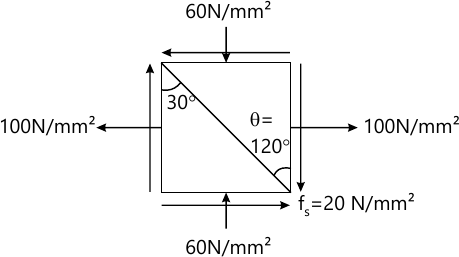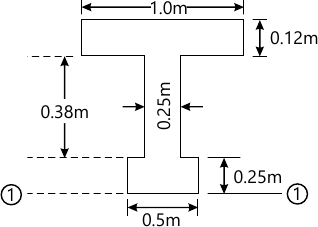MORE IN Strength of Materials
VTU Civil Engineering (Semester 3)
Strength of Materials
June 2015
Total marks: --
Total time: --
INSTRUCTIONS
(1) Assume appropriate data and state your reasons
(2) Marks are given to the right of every question
(3) Draw neat diagrams wherever necessary

1 (a) Define:
i) Stress
ii) Strain
4 M
1 (b) Derive the relation between modulus of rigidity and Young's modulus of Elasticity and define elastic constants.
8 M
1 (c) The modulus of rigidity for a material is 51Gpa. A 10mm diameter rod of the material was subjected to an axial load of 10 kN and the change in diameter was observed to be 3×10-3mm. Calculate the Poisson's ratio and the modulus of elasticity.
8 M

2 (a) A reinforced concrete column 300 mm× 300mm has 4 reinforcement bars of steel each 20mm in diameter. Calculate the safe load column can take if the permissible stress in concrete 5.2 N/mm2 and Esteel/Econcrete =18.
8 M
2 (b) A compound bar made of steel plate 60mm wide and 10mm thick to which the copper plate 60 mm wide and 5mm thick are rigidly connected to each other. The length of the bar is 0.7m. If the temperature is raised by 80°C. Determine the stress in each metal and the change in the length.
take: Es=200 GPa αs=12×10-6/°C
Ecu=100 Gpa αcu=17×10-6/°C.
12 M

3 (a) Derive expressions for principle stresses and their planes for two dimensional stress system.
8 M
3 (b) At a point in a strained material, the state of the stress is as shown in the fig. Q3(b). Calculate the normal and the shearing stress on the plane AC. Also find the principal stresses and their planes. Determine the maximum shear stress and their planes.12 M

4 (a) Define:
i) Shear force
ii) Bending moment
iii) Point of contra flexure.
6 M
4 (b) A beam ABCD, 8m long has supports at 'A' and at 'C' which is 6m from 'A'. The beam carries a UDL of 10 kN/m between 'A' and 'C' at point B a 30kN concentrated load acts 2m from the support A and a point load of 15 kN acts at the free end 'D'. Draw the SFD and BMD giving salient values. Also locate the point of contra-flexure if any.
14 M

5 (a) Derive Bernoulli-Euler bending equation $\dfrac {E}{I} = \dfrac {f}{y} = \dfrac {E} {R}$.
6 M
5 (b) The cross section of a beam is shown in Fig. Q5(b). The shear force on the section is 140 kN. Estimate the shear stresses at various points and plot the shear distribution diagram.14 M

6 (a) Derive the equation $EI \dfrac {d^2y}{dx^2} = M_x$ with usual notations.
6 M
6 (b) A beam of constant C/S 10m long is freely supported at its ends and loaded with 2 loads of 60 kN each at 3m from either end. Find the slope at the support and the deflection under any one load. Take EI constant.
14 M

7 (a) Lis the assumptions made in the theory of pure torsion.
4 M
7 (b) Explain:
i) Polar modulus
ii) Torsional rigidity
iii) Polar moment of inertia.
6 M
7 (c) A solid shaft is to transmit 340 kN-m at 120 rpm. If the shear stress of the material should not exceed 80 MPa. Find the diameter required. What percentage saving in weight would be obtained if this shaft i replaced by a hollow one whose di=0.6 do, the length, material and shear stress remaining same.
10 M

8 (a) Distinguish between short column and long column.
4 M
8 (b) Explain:
i) Effective length of column
ii) Slenderness ratio
8 (c) Determine the Euler's crushing load for a hollow cylindrical cast iron column 150mm external diameter and 20mm thick. If it is hinged at both the ends and 6m long compare this load with the crushing load as given by Rankine's formua. Use the constants; $f_c = 550 Mpa \ \ \alpha=\dfrac {1}{1600} \ \ E=80GPa.$